N     0 ≤ N ≤ 7 CMesh points points < 65000
Interactive Loop subdivision spline surface. Use your fingers or mouse to control the model (hold shift key or use mouse wheel to zoom it). Canvas is matched to your browser window. We need N = 6 subdivisions to get smooth light reflex.

# Loop subdivision spline surface

We use the modified Loop subdivision scheme . It is generalization of the Lane-Riesenfeld algorithm for spline curves
1. we subdivide every edge in two parts and make from every old triangle four new small triangles
2. average all new points with respect to the averaging mask
Po = αPo + β ∑ k=1,n Pk .
3. repeat 1-2 steps N times
4. use evaluation mask to calculate the limit points
5. use tangent masks to calculate normals (shall we use subdivided or limit points here?)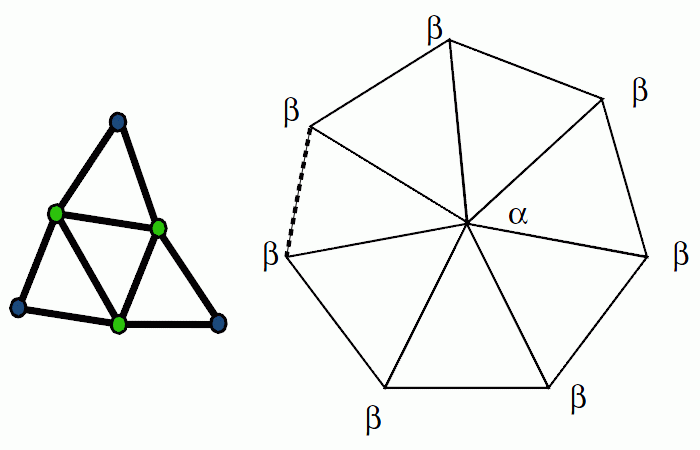Modified averaging mask for a point with n neighbors is 
αnmod = 2 αn - 1,     βn = (1 - αn )/n,
where αn is the Loop averaging mask 
αn = [3/8 + cos(2π/n)/4]2 + 3/8.
Evaluation mask is
εn = 3/(11 - 8αn ).
Two tangents and tangent masks are
Tj = ∑ k=1,n τn,k(j) Pk ,     τn,k(1) = cos(2πk/n),     τn,k(2) = sin(2πk/n)
and the normal direction is N = T1×T2 .

Similar to the Bezier spline patch, we can add per vertex materials and texture UVs as additional dimentions and get colored surfaces (see models below).

## Comments

• The Loop spline normals are not smooth at extraordinary points with 3 neighbor. See also . It is not very important for models. "Octahedron" is almost perfect.
• The script works only with closed, well oriented triangular meshes (without borders).
• The Loop algorithm takes ~200 lines of codes (see loop.js public domain script).

## Loop splines in 3D modelers

3D modeler Art of Illusion supports approximating Loop subdivision splines. Set Edit/Preferences/General/Interactive Surface Error = 0.01 and Approximating Smoothing Method to play with Loop splines. I used it to make the WebGL little dolphin. The AoI model has only 100 vertices. I exported it as Wavefront OBJ file (uncheck Subdivide Smooth Meshes before export). Then converted points and faces from the OBJ into JavaScript arrays by the simple python script
python convert_obj_js.py -i infile.obj -o outfile.js

Blender supports Catmull-Clark splines and Mikael Ohman wrote Stam-Loop subdivision surface modifier WIP draft.

## Loop spline based models

Singular "cone", finger, branch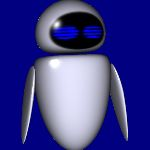EVE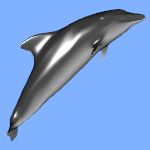dolphin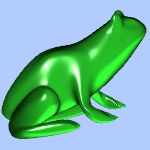frog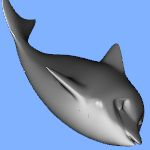little dolphin

Models from the Rome project.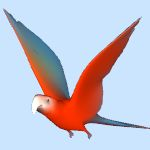parrot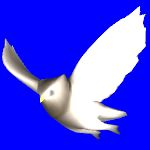owl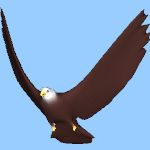eagle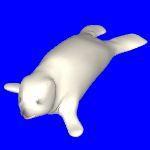seal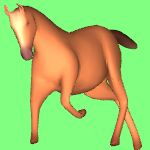horse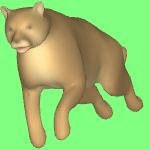lion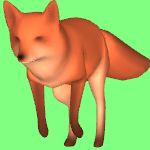fox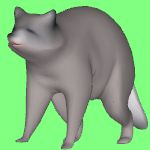raccoon
and flamingo, chow, stork, bunny. In these models colors are blurry a bit due to per face material conversion into per vertex in the scripts (and from textures into per face colors earlier as I think).

See further animated splines.

 Jos Stam & Charles Loop. Quad/Triangle Subdivision EUROGRAPHICS Computer Graphics Forum. November 2003, 22(1). pp. 79-85.
 Charles Loop   Smooth Subdivision Surfaces Based on Triangles M.S. Mathematics Thesis, University of Utah. 1987.
 Hartmut Prautzsch, Georg Umlauf   Improved Triangular Subdivision Schemes. Computer Graphics International 1998: 626-632

Contents   Previous: Subdivision spline curves   Next: Animated splines
updated 18 Apr 2013